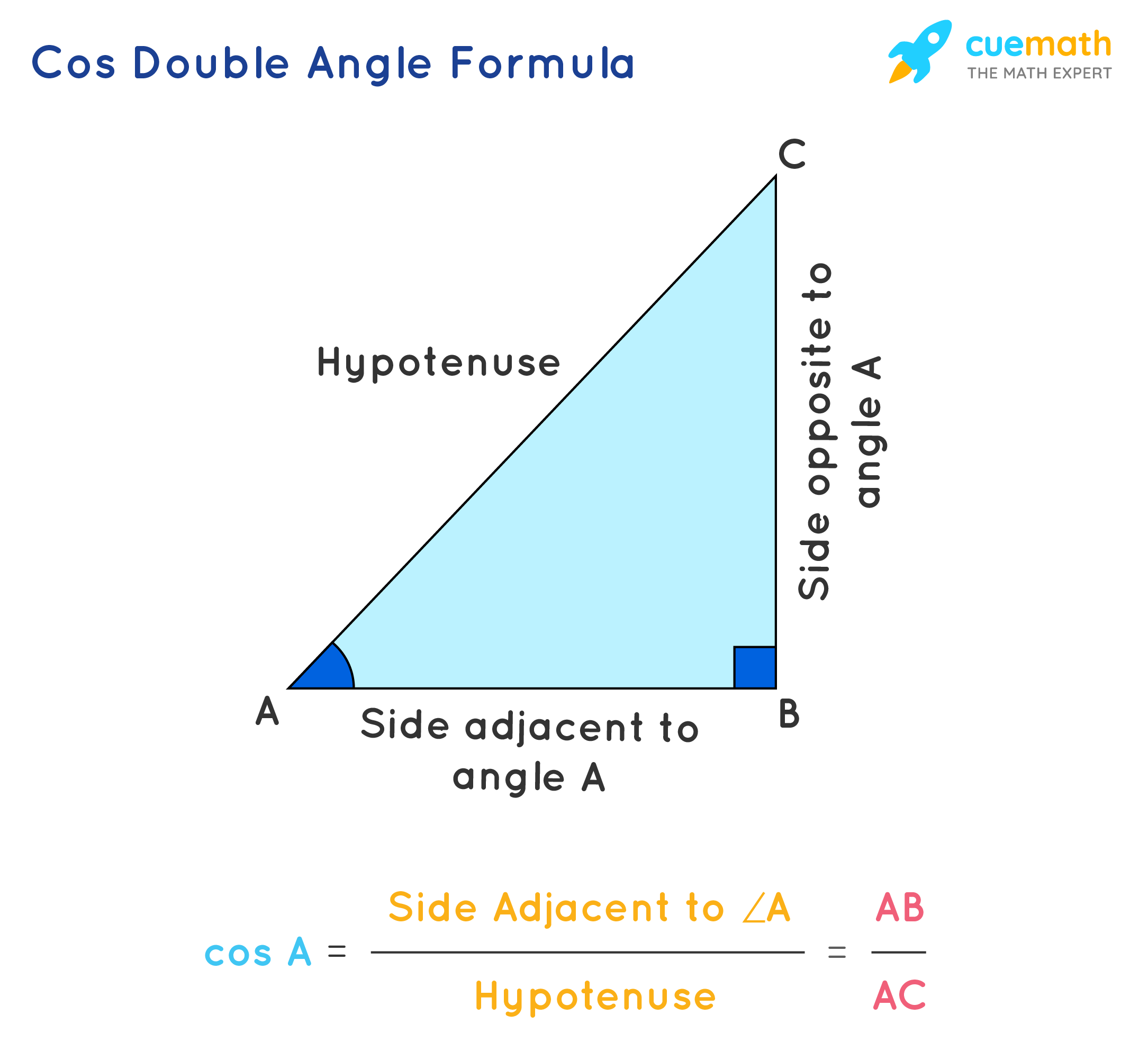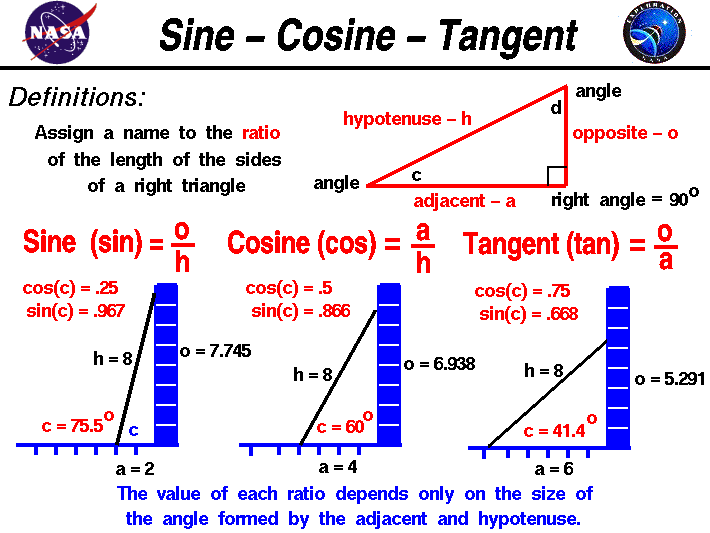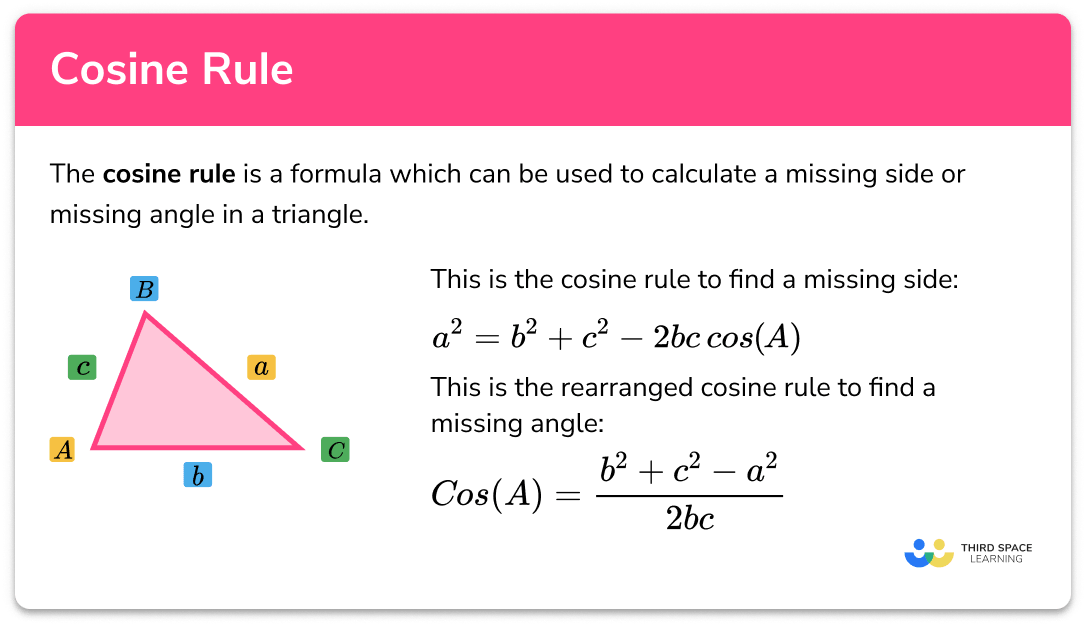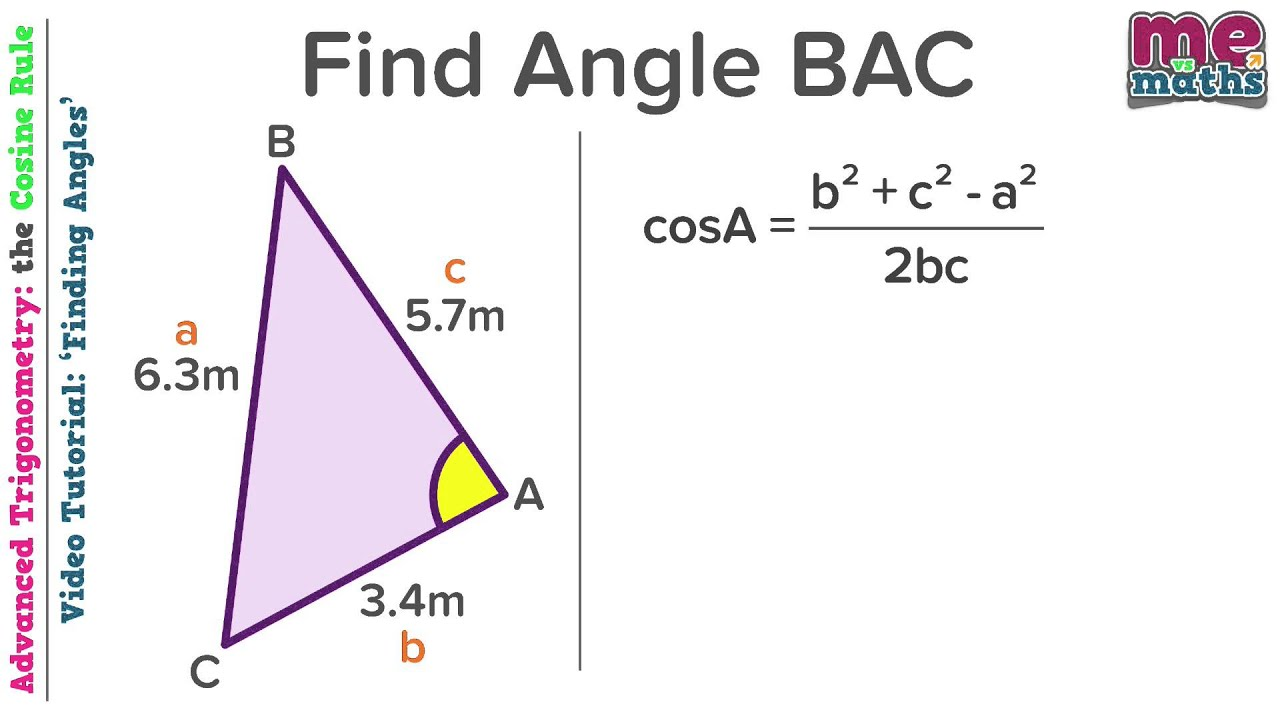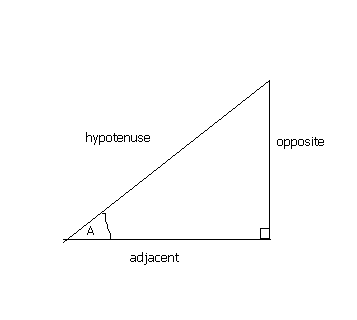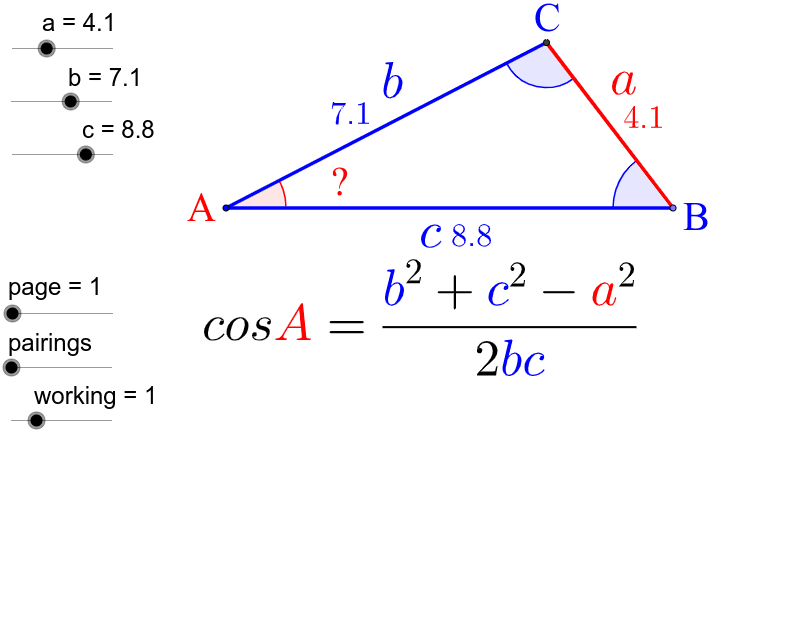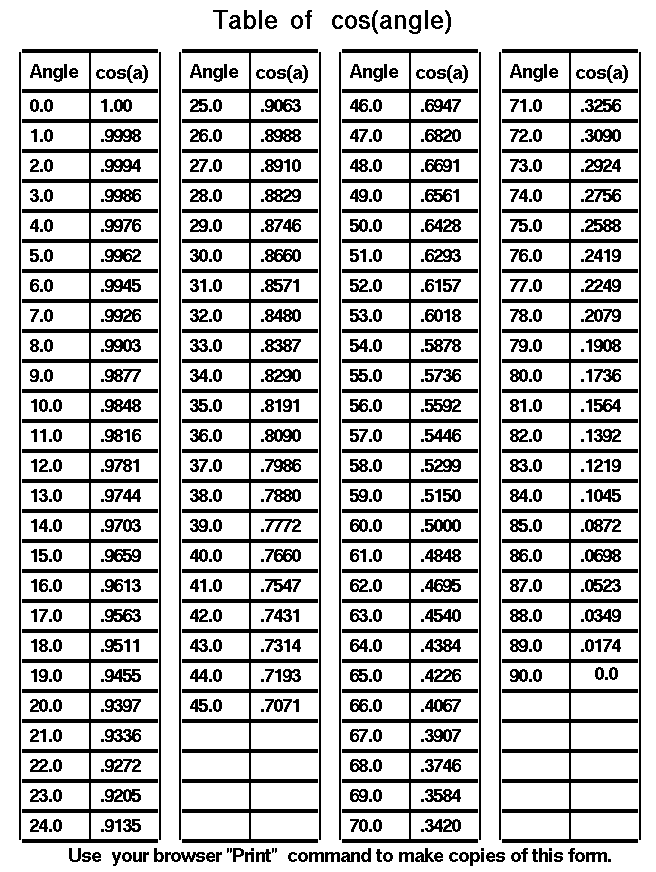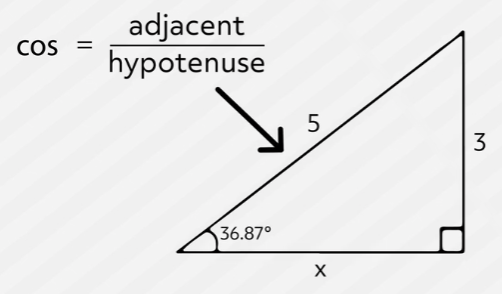# The History of Cosine Function

##### The History of Cos Angle:

Cosine, also known as cos, is a mathematical function that represents the ratio of the adjacent side of a right triangle to its hypotenuse when the angle between them is θ degrees. The concept of trigonometry and cosine have roots in ancient civilization.

 Q: What does cos θ equal? A: Cos θ equals the ratio of the adjacent side of a right triangle to its hypotenuse when the angle between them is θ degrees. Q: How do you calculate cos θ? A: Cos θ can be calculated using a scientific calculator or by using trigonometric tables or formulas. Q: What are some practical applications of cosine functions? A: Cosine functions are used in a variety of fields, including physics, engineering, and astronomy. They can be used to calculate the trajectory of objects in motion, such as projectiles, or to determine the position of celestial objects in the sky.
##### Conclusion:

The cosine function has a rich history that dates back to ancient civilizations. Its usefulness in various fields, from architecture to science and engineering, has made it an essential concept in mathematics. By understanding the function of cosine and its relationship to other trigonometric functions, we can gain a greater appreciation for the fundamental principles that underlie many areas of science and technology.

##### Timeline of Cos Angle:
 Ancient Egypt: Trigonometry was used by Egyptians to build their pyramids. Ancient Greece: Greek mathematician Hipparchus is credited with developing the concept of trigonometry in the second century BC. Middle Ages: During the Islamic Golden Age, Persian mathematician Al-Khwarizmi introduced the concept of sine and cosine functions. 18th century: Swiss mathematician Leonhard Euler formalized the use of trigonometric functions, including cosine, in modern mathematics.
##### Interesting Facts about Cos Angle:
 The cosine function is an example of a periodic function, meaning that it repeats itself at regular intervals. The value of cosine changes as the angle between the adjacent side and hypotenuse changes. In addition to its use in trigonometry, cosine functions are also used in signal processing and Fourier analysis.
##### Image Gallery: